Computational & Technology Resources
an online resource for computational,
engineering & technology publications
Computational Science, Engineering & Technology Series
ISSN 1759-3158
CSETS: 15
INNOVATION IN ENGINEERING COMPUTATIONAL TECHNOLOGY
Edited by: B.H.V. Topping, G. Montero, R. Montenegro
Chapter 18

Fractional Models: Sub and Super-Diffusives, and Undifferentiable Solutions

J.J. Trujillo

Department of Mathematical Analysis, Faculty of Mathematics, University of La Laguna, Tenerife, Spain

Full Bibliographic Reference for this chapter
J.J. Trujillo, "Fractional Models: Sub and Super-Diffusives, and Undifferentiable Solutions", in B.H.V. Topping, G. Montero, R. Montenegro, (Editors), "Innovation in Engineering Computational Technology", Saxe-Coburg Publications, Stirlingshire, UK, Chapter 18, pp 371-401, 2006. doi:10.4203/csets.15.18
Keywords: fractional models, sub-diffusion, anomalous diffusion, fractional derivative, super-diffusion, non-differentiable continuous functions, fractals, generalised Weierstrass functions.

Summary
This paper is dedicated to presenting some aspects of so-called fractional differential equations. In the first part we advance some of the reasons that justify the use of fractional differential equations as an emerging tool for modelling the dynamics of numerous anomalous phenomena, both in nature and in the theory of complex systems.

The main reason behind the usefulness of fractional derivatives in the close link that exists between fractional models and the "Jump" stochastic models, such as the continuous time random walk (CTRW) method, or those of the multiple trapping model type, such as Levy stable distributions and the generalisation of the central limit theorem. Since the second half of the 20th century, the CTRW method has been practically the only tool available to describe sub or super-diffusive phenomena associated with various complex systems. On the other hand, we note that fractional operators allow us to take the memory and non-locality properties of many anomalous processes into account.

In the second part of this paper we briefly present some fractional operators, along with their properties, which will allow us, from a very formal standpoint, to give examples of fractional models with boundary conditions. This should prove helpful in explaining why certain fractional derivative operators are well suited to modelling the dynamics of sub and/or super-diffusive phenomena.

Specifically, consider the well-known uni-dimensional problem associated with, for example, the diffusion of heat in an isolated bar of unit length whose extremities are kept at zero, with a diffusivity constant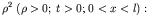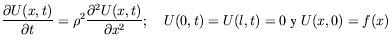We know the solution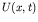is given by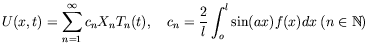(3)

with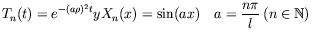(4)

Said solutionclearly varies in time according to: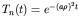. It then decays in time like an ordinary negative exponent. We must then ask: what would we have to do if we wanted the solution to the diffusion problem to proceed in time at an extremely slower rate? What would its analytical differential model be like? One simple approach would be to replace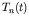with a new function whose exponent decays more slowly. To this end, we can choose either of the two exponential functions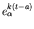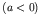or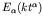, with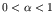. These generalised exponentials are both Mittag-Leffler type functions and they decay more slowly as the parameter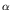approaches 0. Moreover, they have the following properties with respect to their corresponding Riemann-Liouville or Caputo fractional derivative, respectively: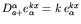and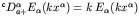. Therefore, the following sub-diffusive models hold: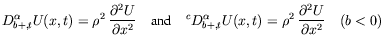However, neither of them can model super-diffusive phenomena since both exponentials stop behaving as a negative exponent when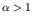. So if we wish to devise a super-diffusive model, we have to find another generalisation of the exponential and another fractional operator. Note that since the generalised Liouville operator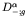has the property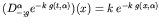with respect to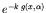, it follows that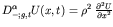is a sub and super-diffusive model. Note that the previous models are easily generalised for the three-dimensional case if, in the second term, the second order spatial derivative is replaced with the corresponding Laplacian.

Lastly, we use some examples based on Weierstrass functions to demonstrate the possibilities fractional models offer for simulating the dynamics of anomalous processes, which classical ordinary models do not.

purchase the full-text of this chapter (price £20)